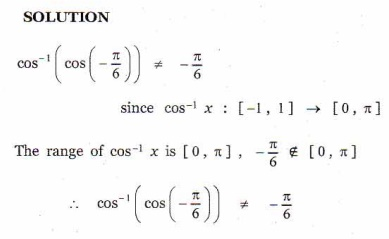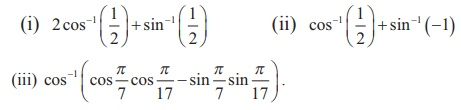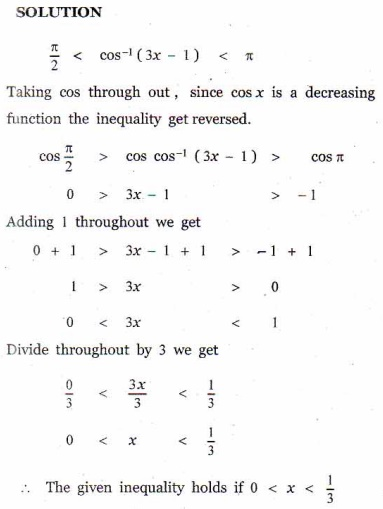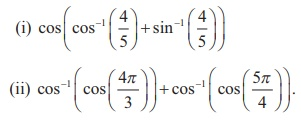Home | | Maths 12th Std | Exercise 4.2: Cosine Function and Inverse Cosine Function

# Exercise 4.2: Cosine Function and Inverse Cosine Function

Maths Book back answers and solution for Exercise questions - Mathematics : Inverse Trigonometric Functions: Cosine Function and Inverse Cosine Function: Exercise Questions with Answer, Solution

EXERCISE 4.2

1. Find all values of such that

(i) -6π ≤ x ≤ 6π and cos x = 0 (ii) -5π ≤ x ≤ 5π and cos x = 1.2. State the reason for4. Find the principal value of cos1 (1/2)5. Find the value of6.Find the domain of7. For what value of x , the inequality π/2 < cos-1 (3x -1) < π holds?8. Find the value of